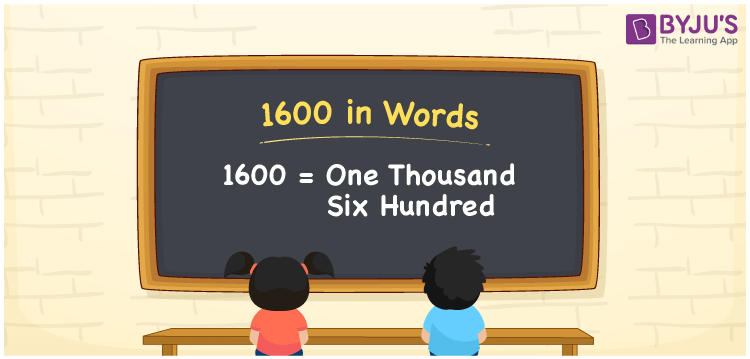# 1600 in Words

1600 in words can be written as One Thousand Six Hundred. Students will be able to learn the conversion of 1600 in words which will help them understand the applications of numbers in our daily lives. If you buy an earphone for Rs. 1600, then you can say that “I have bought an earphone for One Thousand Six Hundred Rupees”. The  number 1600 can be written in words using the English alphabet. The numbers in words can be grasped easily by the students using the resources given at BYJU’S. 1600 in English can be read as “One Thousand Six Hundred”.

 1600 in words One Thousand Six Hundred One Thousand Six Hundred in Numbers 1600

## 1600 in English Words## How to Write 1600 in Words?

Students will learn about the conversion of 1600 into words from place value charts. The number 1600 has four digits. For 1600, the place value chart is prepared in a table form to help students understand it effectively.

 Thousands Hundreds Tens Ones 1 6 0 0

1600 in expanded form is explained in brief here:

1 × Thousand + 6 × Hundred + 0 × Ten + 0 × One

= 1 × 1000  + 6 × 100 + 0 × 10 + 0 × 1

= 1000 + 600

= 1600

= One Thousand Six Hundred

Therefore, 1600 in words is written as One Thousand Six Hundred.

1600 is a natural number that precedes 1601 and succeeds 1599.

1600 in words – One Thousand Six Hundred

Is 1600 an odd number? – No

Is 1600 an even number? – Yes

Is 1600 a perfect square number? – Yes

Is 1600 a perfect cube number? – No

Is 1600 a prime number? – No

Is 1600 a composite number? – Yes

## Frequently Asked Questions on 1600 in Words

Q1

### How do you write 1600 in words?

1600 can be written as “One Thousand Six Hundred” in words.
Q2

### Is 1600 an even number?

1600 is an even number because it is divisible by 2. 1600/2 = 800
Q3

### How can One Thousand Six Hundred be written in numbers?

One Thousand Six Hundred can be written in numbers as 1600.